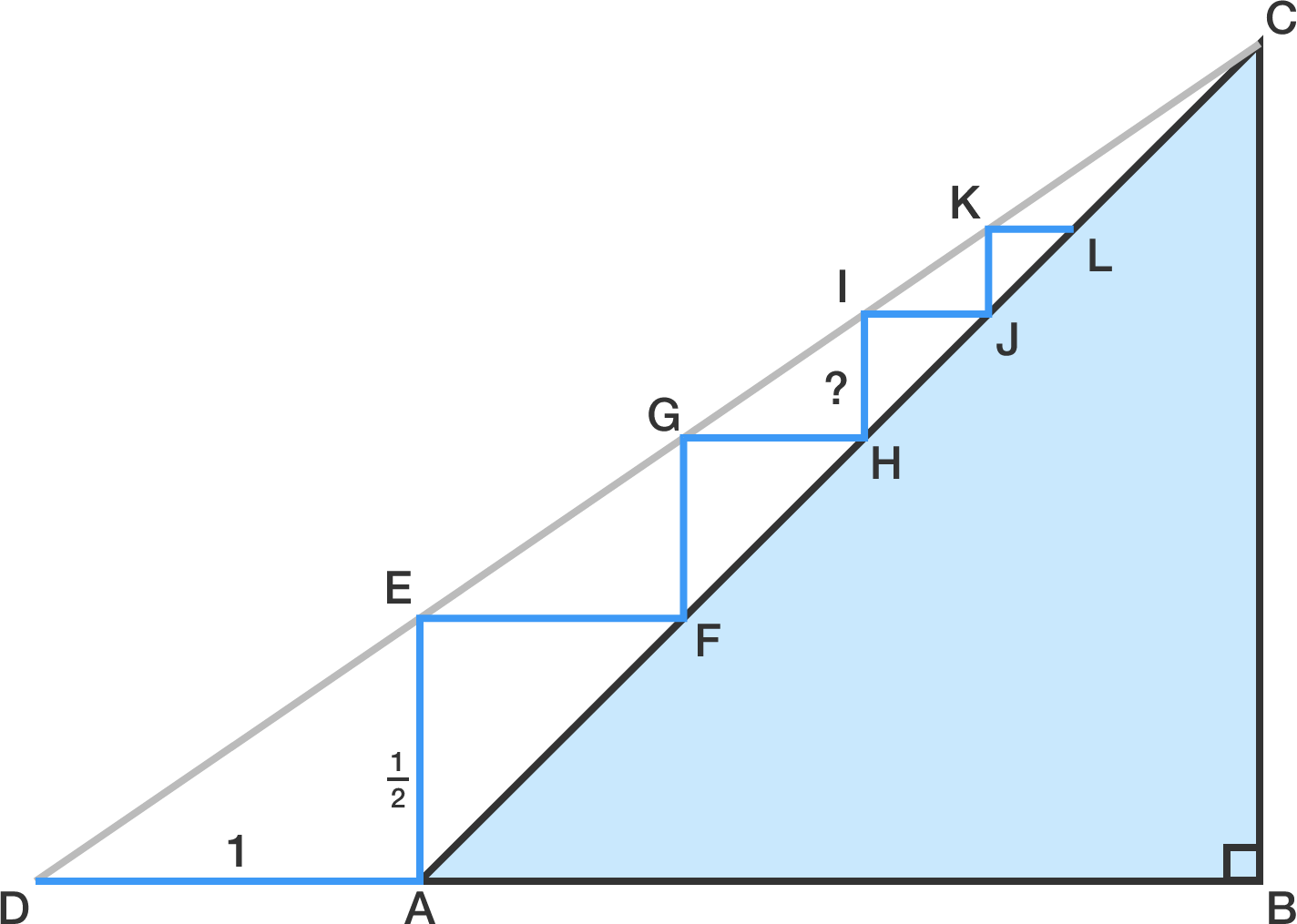# Staircase Heights

Geometry Level 1

An architect is trying to design a short staircase. His plan is shown in the diagram below, where all the vertical and horizontal lines meet at right angles.

In the figure below, $AB = BC$ and $\angle ABC=90^\circ.$ What is the height of the third step $($marked $HI)?$

Note: No variables or calculations are needed to solve this problem.×